# How To use the Node Material

The Node Material is a new material introduced with Babylon.js v4.1. It lets you create a material based on custom shaders but without having to deal with shader code. All the shader creation will be done using either an UI (the Node Material Editor) or by creating and connecting nodes (the Node Material blocks).

## Creating a Node Material using code

### Initial steps

To start using the Node Material, you just need to instantiating one:

``````var nodeMaterial = new BABYLON.NodeMaterial("node material", scene, { emitComments: true });
``````

Please note that the third parameter will contain optional values that will let you configure how the material will build its shader:

• `emitComments`: Set this value to true if you want the blocks to emit comments in the shader code

Blocks can be added by just instantiating them:

``````var morphTargets = new BABYLON.MorphTargetsBlock("morphTargets");
``````

For input blocks, you can then define either their value or the source of the value:

``````var timeInput = new BABYLON.InputBlock("time");
timeInput.value = 0;
``````

or

``````var viewProjectionInput = new BABYLON.InputBlock("viewProjection");
viewProjectionInput.setAsSystemValue(BABYLON.NodeMaterialSystemValues.ViewProjection);
``````

System values can be:

• BABYLON.NodeMaterialSystemValues.World
• BABYLON.NodeMaterialSystemValues.View
• BABYLON.NodeMaterialSystemValues.WorldView
• BABYLON.NodeMaterialSystemValues.Projection
• BABYLON.NodeMaterialSystemValues.ViewProjection
• BABYLON.NodeMaterialSystemValues.WorldViewProjection
• BABYLON.NodeMaterialSystemValues.CameraPosition
• BABYLON.NodeMaterialSystemValues.FogColor
• BABYLON.NodeMaterialSystemValues.DeltaTime

Input blocks can also take their value from a mesh attribute:

``````let positionInput = new BABYLON.InputBlock("position");
positionInput.setAsAttribute("position");
``````

Attributes can be:

• position
• normal
• tangent
• uv
• uv2
• matricesIndices
• matricesWeights
• matricesIndicesExtra
• matricesWeightsExtra

When you manually set the value of an InputNode, you can flag it as `node.isConstant` to indicate that the value will not be dynamically updated and thus the node material will be able to optimize the block by not generating an uniform for this value.

• `isSystemValue`
• `isAttribute`
• `isUniform`
• `isConstant`

When an InputNode is an uniform (eg. a manual value that will be sent to the shader) and not a constant, you can set `inputNode.visibleInInspector` to true so users will be able to visually control the value of the node using Babylon.js Inspector.

You can even csutomize the look and feel of the Inspector UI by defining `inputNode.min` and `inputNode.max` to get a slider instead of an input text box.

### Connecting blocks

By default calling `block.connectTo(otherBlock)` will try to establish a connection by picking an output from the first block and connect it to an available input in the second one:

``````var positionInput = new BABYLON.InputBlock("position");
positionInput.setAsAttribute("position");

var worldInput = new BABYLON.InputBlock("world");
worldInput.setAsSystemValue(BABYLON.NodeMaterialSystemValues.World);

var worldPos = new BABYLON.TransformBlock("worldPos");
positionInput.connectTo(worldPos);
worldInput.connectTo(worldPos);
``````

If you do not want to use the automatic connection you can then pick the output and the input you want to connect directly:

``````worldInput.output.connectTo(boneBlock.world);
``````

You can check if two connection points can connect with

``````if (worldInput.output.canConnectTo(boneBlock.world)) {
...
}
``````

The system will throw an exception if you try to connect two incompatible connection points.

When connected, two connection points can be disconnected with:

``````worldInput.output.disconnectFrom(boneBlock.world);
``````

### Gettings blocks

Once a graph is built inside a NodeMaterial, you can use the following API to get a specific node by name:

``````let block = nodeMaterial.getBlockByName("MyBlock");
``````

You can also get a block using a predicate:

``````let block = nodeMaterial.getBlockByPredicate((b) => b.getClassName() === "AddBlock" && b.name === "foo");
``````

Or you can also use this API to get an InputNode and use it to setup its value if the node is set manual value:

``````let block = nodeMaterial.getInputBlockByPredicate((b) => b.name === "foo");
block.value = 10;
``````

Note that while this API generically works for all input blocks, not all input blocks have a "value" attribute. For example texture blocks would have block.texture instead of block.value. Be sure to check the API documentation for detail.

You can access the list of InputBlocks with:

``````nodeMaterial.getInputBlocks();
``````

Or you can get all blocks registered with a node material with:

``````nodeMaterial.attachedBlocks
``````

Be sure to also check out the full API documentation.

### List of available blocks

By default, the node material provides the following blocks:

• Animation:

• `Bones`: Provides a world matrix for each vertex, based on skeletal (bone/joint) animation.

• Inputs:
• matricesIndices: Vector4
• matricesWeights: Vector4
• matricesIndicesExtra: Vector4
• matricesWeightsExtra: Vector4
• world: Matrix
• Outputs:
• output: Matrix
• `MorphTargets`: Provides the final positions, normals, tangents, and uvs based on morph targets in a mesh.

• Inputs:
• position: Vector3
• normal: Vector3
• tangent: Vector3
• uv: Vector2
• Outputs:
• positionOutput: Vector3
• normalOutput: Vector3
• tangentOutput: Vetor3
• uvOutput: Vector2
• Color Management:

• `Desaturate`: Convert a color input into a grayscale representation.

• Inputs:
• color: Color3
• level: Float
• Outputs:
• output: Color3
• `Gradient`: Returns the color in the gradient represented by the target value of the input.

• Inputs:
• value: Float.
• Outputs:
• output: Color3.
• `Posterize`: Reduces the number of values in each channel to the number in the corresponding channel of steps.

• Inputs:
• value: Float, Vector2, Vector3, Vector4, Color3, or Color4.
• steps: Float, Vector2, Vector3, Vector4, Color3, or Color4.
• Outputs:
• output: Float, Vector2, Vector3, Vector4, Color3, or Color4. Output varies based on input types.
• `ReplaceColor`: Replaces a reference color in value with the color in replacement blended by distance.

• Inputs:
• value: Vector2, Vector3, Vector4, Color3, or Color4.
• reference: Vector2, Vector3, Vector4, Color3, or Color4.
• distance: Float
• replacement: Vector2, Vector3, Vector4, Color3, or Color4.
• Outputs:
• output: Vector2, Vector3, Vector4, Color3, or Color4. Output varies based on input types.
• Conversion:

• `ColorMerger`: Combines float input channels into a color.

• Inputs:
• r: Float
• g: Float
• b: Float
• a: Float
• Outputs:
• rgba: Color4
• rgb: Color3
• `ColorSplitter`: Separates color input channels into individual floats.

• Inputs:
• rgba: Color4
• rgb: Color3
• Outputs:
• rgb: Color3
• r: Float
• g: Float
• b: Float
• a: Float
• `VectorMerger`: Combines up to four input floats into a vector.

• Inputs:
• x: Float
• y: Float
• z: Float
• w: Float
• Outputs:
• xyzw: Vector4
• xyz: Vector3
• xy: Vector2
• `VectorSplitter`: Separates vectors input channels into individual floats.

• Inputs:
• xyzw: Vector4
• xyz: Vector3
• xy: Vector2
• Outputs:
• xyz: Vector3
• xy: Vector2
• x: Float
• y: Float
• z: Float
• w: Float
• Inputs:

• `Color3`: A color made up of red, green, and blue channel values.

• Output:
• output: Color3
• `Color4`: A color made up of red, green, blue, and alpha channel values.

• Output:
• output: Color4
• `DeltaTime`: A float representing the time that has passed since the last frame was rendered.

• Output:
• output: Float
• `Float`: A floating point number representing a value with a fractional component.

• Output:
• output: Float
• `FragCoord`: The gl_FragCoord predefined variable (window relative coordinates (x,y,z,1/w))

• Output:
• xy: Vector2
• xyz: Vector3
• xyzw: Vector4
• x: Float
• y: Float
• z: Float
• w: Float
• `ReflectionTexture`: Creates a reflection from the input texture.

• Input:
• position: Vector3
• worldPosition: Vector4
• worldNormal: Vector4
• world: Matrix
• cameraPosition: Vector3
• view: Matrix
• Output:
• rgb: Color3
• r: Float
• g: Float
• b: Float
• `ScreenSize`: The size of the screen window

• Output:
• xy: Vector2
• x: Float
• y: Float
• `Texture`: A node for reading a linked or embedded texture file.

• Inputs:
• uv: Vector2 (mesh.uv automatically attached).
• Outputs:
• rgba: Vector4
• rgb: Vector3
• r: Float
• g: Float
• b: Float
• a: Float
• `Time`: A float value that represents the time that has passed since the scene was loaded.

• Output:
• output: Float
• `Vector2`: a vector composed of X and Y channels.

• Output:
• output: Vector2
• `Vector3`: a vector composed of X, Y, and Z channels.

• Output:
• output: Vector3
• `Vector4`: a vector composed of X, Y, Z, and W channels.

• Output:
• output: Vector4
• Interpolation:

• `Lerp`: Outputs a value that is a mix of the left and right inputs based on the target value.

• Inputs:
• left: Float, Vector2, Vector3, Vector4, Color3, or Color4.
• right: Float, Vector2, Vector3, Vector4, Color3, or Color4
• Output:
• output: Float, Vector2, Vector3, Vector4, Color3, or Color4. Output varies based on left and right input types.
• `NLerp`: Outputs a value that is a mix of the left and right inputs based on the target's normalized value.

• Inputs:
• left: Float, Vector2, Vector3, Vector4, Color3, or Color4.
• right: Float, Vector2, Vector3, Vector4, Color3, or Color4
• Output:
• output: Float, Vector2, Vector3, Vector4, Color3, or Color4. Output varies based on left and right input types.
• `SmoothStep`: Outputs a value based on a the input value's position on a curve between the two edge values.

• Inputs:
• value: Float
• edge0: Float
• edge1: Float
• Output:
• output: Float
• `Step`: Outputs 1 for any input value above the edge input, outputs 0 for any input value below the edge input.

• Input:
• value: Float
• edge: Float
• Output:
• output: Float
• Math: Standard:

• `Add`: Adds the left and right inputs of the same type together.

• Inputs:
• left: Float, Vector2, Vector3, Vector4, Color3, or Color4.
• right: Float, Vector2, Vector3, Vector4, Color3, or Color4.
• Outputs:
• output: Float, Vector2, Vector3, Vector4, Color3, or Color4. Output varies based on input types.
• `Divide`: Divides the left input by the right input of the same type.

• Inputs:
• left: Float, Vector2, Vector3, Vector4, Color3, or Color4.
• right: Float, Vector2, Vector3, Vector4, Color3, or Color4.
• Outputs:
• output: Float, Vector2, Vector3, Vector4, Color3, or Color4. Output varies based on input types.
• `Max`: Outputs the largest value between the left and right inputs of the same type.

• Inputs:
• left: Float, Vector2, Vector3, Vector4, Color3, or Color4.
• right: Float, Vector2, Vector3, Vector4, Color3, or Color4.
• Outputs:
• output: Float, Vector2, Vector3, Vector4, Color3, or Color4. Output varies based on input types.
• `Min`: Outputs the smallest value between the left and right inputs of the same type.

• Inputs:
• left: Float, Vector2, Vector3, Vector4, Color3, or Color4.
• right: Float, Vector2, Vector3, Vector4, Color3, or Color4.
• Outputs:
• output: Float, Vector2, Vector3, Vector4, Color3, or Color4. Output varies based on input types.
• `Mod`: Outputs the value of the left input modulo the right input

• Inputs:
• left: Float, Vector2, Vector3, Vector4, Color3, or Color4.
• right: Float, Vector2, Vector3, Vector4, Color3, or Color4.
• Outputs:
• output: Float, Vector2, Vector3, Vector4, Color3, or Color4. Output varies based on input types.
• `Multiply`: Multiplies the left and right inputs of the same type together.

• Inputs:
• left: Float, Vector2, Vector3, Vector4, Color3, or Color4.
• right: Float, Vector2, Vector3, Vector4, Color3, or Color4.
• Outputs:
• output: Float, Vector2, Vector3, Vector4, Color3, or Color4. Output varies based on input types.
• `Negate`: Multiplies the input by -1.

• Inputs:
• left: Float, Vector2, Vector3, Vector4, Color3, or Color4.
• right: Float, Vector2, Vector3, Vector4, Color3, or Color4.
• Outputs:
• output: Float, Vector2, Vector3, Vector4, Color3, or Color4. Output varies based on input types.
• `OneMinus`: Subtracts each channel of the input value from 1 (1 - input).

• Inputs:
• left: Float, Vector2, Vector3, Vector4, Color3, or Color4.
• right: Float, Vector2, Vector3, Vector4, Color3, or Color4.
• Outputs:
• output: Float, Vector2, Vector3, Vector4, Color3, or Color4. Output varies based on input types.
• `Reciprocal`: Quotient of 1 divided by the input.

• Inputs:
• left: Float, Vector2, Vector3, Vector4, Color3, or Color4.
• right: Float, Vector2, Vector3, Vector4, Color3, or Color4.
• Outputs:
• output: Float, Vector2, Vector3, Vector4, Color3, or Color4. Output varies based on input types.
• `Scale`: Multiplies the input channels by a float factor.

• Inputs:
• Input: Float, Vector2, Vector3, Vector4, Color3, or Color4.
• Factor: Float
• Output:
• output: Float, Vector2, Vector3, Vector4, Color3, or Color4. Output varies based on input types.
• `Sign`: Returns 1 if the input is positive, 0 if input is equal to 0, or -1 if the input is negative.

• Input:
• input: Float
• Output:
• output: Float
• `Sqrt`: Outputs the the square root of the input value.

• Input:
• input: Float
• Output:
• output: Float
• `Subtract`: Subtracts the right input from the left input of the same type.

• Inputs:
• left: Float, Vector2, Vector3, Vector4, Color3, or Color4.
• right: Float, Vector2, Vector3, Vector4, Color3, or Color4.
• Outputs:
• output: Float, Vector2, Vector3, Vector4, Color3, or Color4. Output varies based on input types.
• Math: Scientific:

• `Abs`: Outputs the absolute value of the input value.

• Input:
• input: Float
• Output:
• output: Float
• `ArcCos`: Outputs the inverse of the cosine value based on the input value.

• Input:
• input: Float
• Output:
• output: Float
• `ArcSin`: Outputs the inverse of the sine value based on the input value.

• Input:
• input: Float
• Output:
• output: Float
• `ArcTan`: Outputs the inverse of the tangent value based on the input value.

• Input:
• input: Float
• Output:
• output: Float
• `ArcTan2`: Outputs the inverse of the tangent value based on the input value.

• Input:
• input: Float
• Output:
• output: Float
• `Cos`: Outputs the cosine value based on the input value.

• Input:
• input: Float
• Output:
• output: Float
• `DegreesToRadians`: Converts the input degrees value to radians.

• Input:
• input: Float
• Output:
• output: Float
• `Exp`: Outputs the input value multiplied by itself 9 time. (Exponent of 10)

• Input:
• input: Float
• Output:
• output: Float
• `Exp2`: Outputs the input value multiplied by itself 1 time. (Exponent of 2)

• Input:
• input: Float
• Output:
• output: Float
• `Fract`: Outputs only the fractional value of a floating point number.

• Input:
• input: Float
• Output:
• output: Float
• `Log`: The logarithmic value based on the input value.

• Input:
• input: Float
• Output:
• output: Float
• `Pow`: Outputs the input value multiplied by itself the number of times equal to the power input (Exponent of power)

• Input:
• value: Float
• power: Float
• Output:
• output: Float
• `RadiansToDegrees`: Converts the input radians value to degrees.

• Input:
• input: Float
• Output:
• output: Float
• `SawToothWave`: Outputs a sawtooth pattern value between -1 and 1 based on the input value.

• Input:
• input: Float
• Output:
• output: Float
• `Sin`: Outputs the the sine value based on the input value.

• Input:
• input: Float
• Output:
• output: Float
• `SquareWave`: Outputs a stepped pattern value between -1 and 1 based on the input value.

• Input:
• input: Float
• Output:
• output: Float
• `Tan`: Outputs the the tangent value based on the input value.

• Input:
• input: Float
• Output:
• output: Float
• `TriangleWave`: Outputs a sawtooth pattern value between 0 and 1 based on the input value.

• Input:
• input: Float
• Output:
• output: Float
• Math: Vector:

• `Cross`: Outputs a vector that is perpendicular to two input vectors.

• Inputs:
• left: Vector2, Vector3, Vector4, Color3, or Color4.
• right: Vector2, Vector3, Vector4, Color3, or Color4.
• Output:
• output: Vector2, Vector3, Vector4, Color3, or Color4. Output varies based on left and right input types.
• `Derivative`: FRAGMENT SHADER ONLY. Provides the rate of change for an input on a given axis (x,y).

• Inputs:
• input: Float, Vector2, Vector3, Vector4, Color3, or Color4.
• Output:
• dx: Float, Vector2, Vector3, Vector4, Color3, or Color4. Output varies based on left and right input types.
• dy: Float, Vector2, Vector3, Vector4, Color3, or Color4. Output varies based on left and right input types.
• `Distance`: Provides a distance vector based on the left and right input vectors.

• Inputs:
• left: Vector2, Vector3, Vector4, Color3, or Color4.
• right: Vector2, Vector3, Vector4, Color3, or Color4.
• Outputs:
• output: Vector2, Vector3, Vector4, Color3, or Color4. Output varies based on input types.
• `Dot`: Outputs the cos of the angle between two vectors.

• Inputs:
• left: Vector2, Vector3, Vector4, Color3, or Color4.
• right: Vector2, Vector3, Vector4, Color3, or Color4.
• Output:
• output: Float
• `Fresnel`: Outputs the grazing angle of the surface of the mesh, relative to a camera influenced by the bias and power inputs.

• Input:
• worldNormal: Vector4
• viewDirection: Vector3
• bias: Float
• power: Float
• Output:
• fresnel: Float
• `Length`: Outputs the length of an input vector.

• Inputs:
• left: Vector2, Vector3, Vector4, Color3, or Color4.
• right: Vector2, Vector3, Vector4, Color3, or Color4.
• Outputs:
• output: Vector2, Vector3, Vector4, Color3, or Color4. Output varies based on input types.
• `Reflect`: Outputs the direction of the input vector reflected across the surface normal.

• Inputs:
• incident: Vector3
• normal: Vector3
• Outputs:
• output: Vector3
• `Refract`: Outputs a direction simulating a deflection of the input vector.

• Inputs:
• incident: Vector3
• normal: Vector3
• ior: Float
• Outputs:
• output: Vector3
• `Rotate2D`: Rotates UV coordinates around the W axis.

• Inputs:
• input: Vector2
• angle: Float
• Outputs:
• output: Vector2
• `Transform`: Transforms a input vector based on the input matrix.

• Inputs:
• vector: Vector2, Vector3, Vector4, Color3, or Color4
• transform: Matrix
• Output:
• output: Vector4
• Matrices:

• `Matrix`: A 4x4 table of related values.

• Output:
• output: Matrix
• `ProjectionMatrix`: A matrix to remap points in 3D space to 2D plane relative to the screen.

• Output:
• output: Matrix
• `ViewMatrix`: A matrix to remap points in 3D space to 2D plane relative to the view of the scene camera.

• Output:
• output: Matrix
• `ViewProjectionMatrix`: A matrix to remap points in 3D space to 2D view space before remapping to 2D screen space.

• Output:
• output: Matrix
• `WorldMatrix`: A matrix to remap points in 3D local space to 3D world space.

• Output:
• output: Matrix
• `WorldViewMatrix`: A matrix to remap points in 3D local space to 3D world space, and ending in 2D camera space.

• Output:
• output: Matrix
• `WorldViewProjectionMatrix`: A matrix to remap points in 3D local space to 3D world space, then to 2D camera space, and ending in 2D screen space.

• Output:
• output: Matrix
• Mesh:

• `Color`: Outputs the RGBA color of each vertex in the mesh.

• Output:
• output: Color4
• `FrontFacing`: Returns 1 if a mesh triangle faces the normal direction and 0 if it does not.

• Outputs:
• output: Float
• `Instances`: Provides the world matrix for each instance to apply this material to all instances.

• Inputs:
• world0: Vector4
• world1: Vector4
• world2: Vector4
• world3: Vector4
• world: worldMatrix
• Output:
• output: Matrix
• `MatricesIndices`: A Vector4 representing the vertex to bone skinning assignments.

• Output:
• output: Vector4
• `MatricesWeights`: A Vector4 representing the vertex to bone skinning weights.

• Output:
• output: Vector4
• `Normal`: A Vector3 representing the normal of each vertex of the attached mesh.

• Output:
• output: Vector3
• `NormalBlend`: Outputs the result of blending two normal maps together using a per-channel screen.

• Input:
• normalMap0: Vector3
• normalMap1: Vector3
• Output:
• output: Vector3
• `PerturbNormal`: Creates high-frequency detail normal vectors based on a normal map, the world position, and world normal.

• Input:
• worldPosition: Vector4
• worldNormal: Vector4
• uv: Vector2
• normalMap: Color3
• strength: Float
• Output:
• output: Vector4
• `Position`: A Vector3 representing the position of each vertex of the attached mesh.

• Output:
• output: Vector3
• `Tangent`: A Vector3 representing the tangent of each vertex of the attached mesh.

• Output:
• output: Vector3
• `UV`: A Vector2 representing the UV coordinates of each vertex of the attached mesh.

• Output:
• output: Vector2
• `WorldNormal`: A Vector4 representing the normal of each vertex of the attached mesh transformed into world space.

• Input:
• vector: Vector3
• transform: Matrix
• Output:
• output: Vector4
• `WorldPosition`: A Vector4 representing the position of each vertex of the attached mesh transformed into world space.

• Input:
• vector: Vector3
• transform: Matrix
• Output:
• output: Vector4
• Noises:

• `RandomNumber`: Provides a random number based on an input seed.

• Inputs:
• seed: Vector2
• Output:
• output: Float
• `SimplexPerlin3D`: Creates a type of gradient noise with few directional artifacts.

• Inputs:
• seed: Vector3
• Output:
• output: Float
• `WorleyNoise3D`: Creates a random pattern resembling cells.

• Inputs:
• seed: Vector3
• jitter: Float
• Output:
• output: Vector2
• Outputs:

• `Discard`: A final node that will not output a pixel below the cutoff value.

• Inputs:
• value: Float
• cutoff: Float
• `FragmentOutput`: A mandatory final node for outputing the color of each pixel.

• Inputs:
• rgba: Vector4
• rgb: Vector3
• a: Float
• `VertexOutput`: A mandatory final node for outputing the position of each vertex.

• Inputs:
• vector: Vector4
• Particle:

• `ParticleBlendMultiply`: The "blend/multiply" module of the particle shader

• Inputs:
• color: Color4
• alphaTexture: Float
• alphaColor: Float
• Outputs:
• blendColor: Color4
• `ParticleColor`: The color of the particle

• Outputs:
• output: Color4
• `ParticlePositionWorld`: The world position of the particle

• Outputs:
• output: Vector3
• `ParticleRampGradient`: The "ramp gradient" module of the particle shader

• Inputs:
• color: Color4
• Outputs:
• rampColor: Color4
• `ParticleTexture`: The texture of the particle

• Inputs:
• uv: Vector2
• Outputs:
• rgba: Color4
• rgb: Color3
• r: Float
• g: Float
• b: Float
• a: Float
• `ParticleTextureMask`: The textureMask property of the particle

• Outputs:
• output: Color4
• `ParticleUV`: the uv coordinates of the particle

• Outputs:
• output: Vector2
• PBR: (since 4.2)

• `Anisotropy`: The anisotropy module of the PBR material

• Inputs:
• intensity: Float
• direction: Vector2 - note that if you read the direction from a texture, you will probably want to apply the transformation `texture.xy * 2 - 1` to get coordinates between -1 and 1!
• uv: Vector2
• worldTangent: Vector4
• Outputs:
• anisotropy: can only be used as input of the `PBRMetallicRoughness` block
• `ClearCoat`: The clear coat module of the PBR material

• Inputs:
• intensity: Float
• roughness: Float
• indexOfRefraction: Float
• normalMapColor: Color3
• uv: Vector2
• tintColor: Color3
• tintAtDistance: Float
• tintThickness: Float
• worldTangent: Vector4
• Outputs:
• clearcoat: can only be used as input of the `PBRMetallicRoughness` block
• `PBRMetallicRoughness`: The PBR material implementing the metallic/roughness model

• Inputs:
• worldPosition: Vector4
• worldNormal: Vector4
• view: Matrix
• cameraPosition: Vector3
• perturbedNormal: Vector4
• baseColor: Color3
• metallic: Float
• roughness: Float
• ambientOcc: Float
• opacity: Float
• indexOfRefraction: Float
• ambientColor: Color3
• reflection: output of the `Reflection` block
• clearcoat: output of the `ClearCoat` block
• sheen: output of the `Sheen` block
• subsurface: output of the `SubSurface` block
• anisotropy: output of the `Anisotropy` block
• Outputs:
• ambientClr: Color3
• diffuseDir: Color3
• specularDir: Color3
• clearcoatDir: Color3
• sheenDir: Color3
• diffuseInd: Color3
• specularInd: Color3
• clearcoatInd: Color3
• sheenInd: Color3
• refraction: Color3
• lighting: Color3
• alpha: Float

Notes:

• The `Dir` suffix in the name of the outputs means `Direct` and are components from direct lighting (spot, point light, directional, ...).
• The `Ind` suffix in the name of the outputs means `Direct` and are components from indirect lighting (IBL, ...).
• The `lighting` output is the combination (sum) of all the other outputs (except for `shadow` and `alpha`) and is the one to use if you want to deal with the final output color. This color is in gamma space.
• All the `XXXClr`, `XXXDir` and `XXXInd` outputs are individual lighting components and can be used if you need to perform further processing with them. These components are in linear space.
• The `shadow` output is the shadow component when this material is used for shadow rendering (0 means completely in shadow from all lights and 1 means fully visible by all lights). You can use it for additional special effects (see https://playground.babylonjs.com/#Y642I8 -
for tinted shadows for eg - it's for the standard material but it would work the same for PBR too) but note that you don't need to do anything with it by default to have shadows rendered correctly for your material
• `Reflection`: The reflection module of the PBR material

• Inputs:
• position: Vector3
• world: Matrix
• color: Color3
• Outputs:
• reflection: can only be used as input of the `PBRMetallicRoughness` block
• `Refraction`: The refraction module of the PBR material (used by the `SubSurface` block)

• Inputs:
• intensity: Float
• tintAtDistance: Float
• Outputs:
• refraction: can only be used as input of the `SubSurface` block
• `Sheen`: The sheen module of the PBR material

• Inputs:
• intensity: Float
• color: Color3
• roughness: Float
• Outputs:
• sheen: can only be used as input of the `PBRMetallicRoughness` block
• `SubSurface`: The sub surface module of the PBR material

• Inputs:
• thickness: Float
• tintColor: Color3
• translucencyIntensity: Float
• translucencyDiffusionDist: Color3
• refraction: output of the `Refraction` block
• Outputs:
• subsurface: can only be used as input of the `PBRMetallicRoughness` block
• PostProcess:

• `CurrentScreen`: The current screen (texture) used to render the post process

• Inputs:
• uv: Vector2
• Outputs:
• rgba: Color4
• rgb: Color3
• r: Float
• g: Float
• b: Float
• a: Float
• `Position2D`: The 2D clip coordinates (values between -1 and 1 for both x and y)

• Outputs:
• output: Vector2
• Range:

• `Clamp`: Outputs values above the maximum or below minimum as maximum or minimum values respectively.

• Input:
• input: Float
• Output:
• input: Float
• `Normalize`: Remaps the length of a vector or color to 1.

• Inputs:
• input: Vector2, Vector3, Vector4, Color3, or Color4.
• Outputs:
• output: Vector2, Vector3, Vector4, Color3, or Color4. Output varies based on input types.
• `Remap`: Remaps input value between sourceMin and sourceMax to a new range between targetMin and targetMax.

• Inputs:
• input: Float, Vector2, Vector3, Vector4, Color3, Color4.
• sourceMin: Float
• sourceMax: Float
• targetMin: Float
• targetMax: Float
• Outputs:
• output: Float, Vector2, Vector3, Vector4, Color3, Color4. Output varies based on input type.
• Round:

• `Ceiling`: Outputs fractional values as the next higher whole number.

• Input:
• input: Float
• Output:
• output: Float
• `Floor`: Outputs fractional values as the next lower whole number.

• Input:
• input: Float
• Output:
• output: Float
• `Round`: Outputs fractional values rounded to the nearest whole number.

• Input:
• input: Float
• Output:
• output: Float
• Scene Attributes:

• `CameraPosition`: Outputs a Vector3 position of the active scene camera.

• Output:
• output: Vector3
• `Fog`: Applies fog to the scene with an increasing opacity based on distance from the camera.

• Input:
• worldPosition: Vector4
• view: viewMatrix (Matrix)
• input: Vector3
• fogColor: Color3
• Output:
• output: Color3
• `FogColor`: The system value for fog color pulled from the scene.

• Output:
• output: Color3
• `ImageProcessing`: Provides access to all of the Babylon image processing properties https://doc.babylonjs.com/how_to/how_to_use_postprocesses

• Input:
• color: Color4
• Output:
• output: Color4
• `Light`: Outputs diffuse and specular contributions from one or more scene lights.

• Input:
• worldPosition: Vector4
• worldNormal: Vector4
• cameraPosition: Vector3
• glossiness: Float
• glossPower: Float
• diffuseColor: Color3
• specularColor: Color3
• Output:
• diffuseOutput: Color3
• specularOutput: Color3
• `LightInformation`: Provides the direction, color and intensity of a selected light based on its world position.

• Input:
• worldPosition: Vector4
• Output:
• direction: Vector3
• color: Color3
• intensity: Float
• `ViewDirection`: Outputs the direction vector of where the camera is aimed.

• Input:
• worldPosition: Vector4
• cameraPosition: Vector3
• Output:
• output: Vector3

### Building the Node Material

Once setup, you can ask the Node Material to build its internal shaders (vertex and fragment) by calling `nodeMaterial.build(true)`. You can set the boolean parameter to true to get a log of the final shaders on the console.

The build function will throw an exception if the shaders cannot be compiled:

``````try {
nodeMaterial.build(true);
} catch (err) {
console.log("Unable to compile because " + err);
}

``````

Once successfully built, you can use the Node Material like any other materials:

``````myMesh.material = nodeMaterial;
``````

### Example

Here is one of the simplest code using the Node Material:

``````var nodeMaterial = new BABYLON.NodeMaterial("node material", scene, { emitComments: true });
var positionInput = new BABYLON.InputBlock("position");
positionInput.setAsAttribute("position");

var worldInput = new BABYLON.InputBlock("world");
worldInput.setAsSystemValue(BABYLON.NodeMaterialSystemValues.World);

var worldPos = new BABYLON.TransformBlock("worldPos");
positionInput.connectTo(worldPos);
worldInput.connectTo(worldPos);

var viewProjectionInput = new BABYLON.InputBlock("viewProjection");
viewProjectionInput.setAsSystemValue(BABYLON.NodeMaterialSystemValues.ViewProjection);

var worldPosdMultipliedByViewProjection = new BABYLON.TransformBlock("worldPos * viewProjectionTransform");
worldPos.connectTo(worldPosdMultipliedByViewProjection);
viewProjectionInput.connectTo(worldPosdMultipliedByViewProjection);

var vertexOutput = new BABYLON.VertexOutputBlock("vertexOutput");
worldPosdMultipliedByViewProjection.connectTo(vertexOutput);

// Pixel
var pixelColor = new BABYLON.InputBlock("color");
pixelColor.value = new BABYLON.Color4(0.8, 0.8, 0.8, 1);

var fragmentOutput = new BABYLON.FragmentOutputBlock("fragmentOutput");
pixelColor.connectTo(fragmentOutput);

``````

Please note that this code is equivalent to:

``````var nodeMaterial = new BABYLON.NodeMaterial("node material", scene, { emitComments: true });
nodeMaterial.setToDefault();
``````

## Using the Node Material Editor

The Node Material Editor can be used to visually edit / build your Node Material.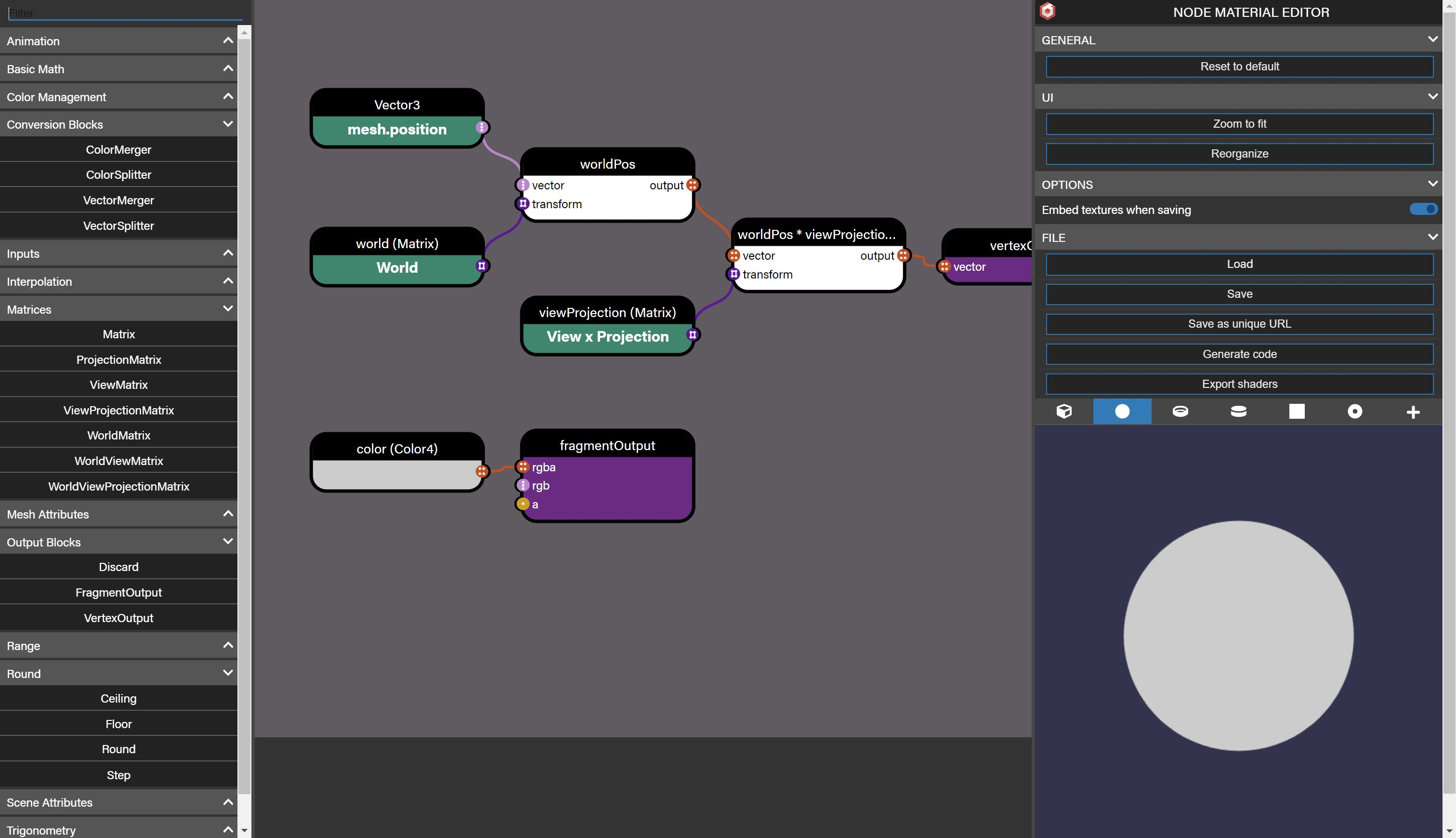To invoke the editor you can call `nodematerial.edit()` but this code must be called inside a user interaction (like a click event). You can also call it through the Inspector:

``````scene.debugLayer.show();
scene.debugLayer.select(nodeMaterial);
``````

When selected in the Inspector, you can find an edit button in the Node Material property pane.

You can also use a standalone version of the editor on https://nme.babylonjs.com

## Recreating the StandardMaterial

As a training exercise and to show what is possible to do with the Node Material Editor, the `StandardMaterial` has been recreated in the NME:

Note that the only difference between the full material and the material without alpha support is that nothing is wire to the `fragmentOutput.a` input. If you don't need alpha support, you should use the "non alpha" node material as alpha-based materials have some constraints:

• they don't write to the zbuffer and are only sorted among themselves, so some sorting rendering artifacts can arise
• they need the `transparencyShadow` property to be `true` for shadow rendering

Let's see how the material has been created and how to use it.

### Main building frames

The material is divided into several frames, mirroring the main features of the standard material:

• Instances
• Morphs and bones
• Ambient
• Diffuse
• Specular
• Reflection
• Emissive
• Bump (normal map)
• Opacity
• Lightmap
• Vertex color
• Fog

In each of these frames, you generally find a boolean float node that enable/disable the feature, and possibly some other properties to fine-tune the feature. Most of these properties are Constant properties, meaning they won't consume a uniform in the shaders and won't be visible in the Inspector / be updatable in javascript: you must change their value directly in the material (they correspond to the `#define` you can find in the standard material shader code).

Note that you won't find this enable/disable property in the Instances, Morphs and bones and Fog frames: they are always enabled. That's because they depend on the mesh geometry / settings (or on a scene setting for Fog): those frames will be a simple "pass-through" if the corresponding feature doesn't exist on the mesh / scene, so no need to explicitly disable it in that case.

There are a number of additional frames that help organizing the graph more cleanly:

• Final normal. This frame takes the output from the Bump frame and builds the final world normal used in subsequent computations (Reflection and Lights). You can change the `TWOSIDEDLIGHTING` boolean if you want the lighting to be applied whatever the triangle side facing are.
• Final diffuse computation. It is the frame responsible for computing the final diffuse component, taking into account the ambient, emissive and vertex color components. Here you can modify the `LINKEMISSIVEWITHDIFFUSE` and `EMISSIVEASILLUMINATION` booleans to change the way the diffuse value is computed.
• Final color computation. Everything is brought together to compute the final rgb color: ambient (texture), specular, reflection and emissive.
• Final alpha computation. After the opacity (alpha) is generated from the Opacity frame, a number of additional computation is performed to produce the final alpha value. You can step in this computation by mean of two booleans, `REFLECTIONOVERALPHA` and `SPECULAROVERALPHA`.
• Premultiply alpha to color. This one does what its title says and is enabled by the `PREMULTIPLYALPHA` boolean.

### Construction notes

The material itself is not so complicated as each feature is generally restricted to its own frame and has few connections with other frames. That helps to keep each building block manageable and easily understandable.

Below are a few things of note.

#### Working without a `if` statement

As you may know, there's no `if` statement / block in the node material editor, so one must be creative to overcome this. Luckily, the standard material does not use this statement heavily (as it's better to avoid it for performance sake), so it is easy enough to deal with it. Most of the time, it is something like `if boolean is true, use this value in subsequent computation, else use that other value instead`. A Lerp block is the tool to use:

``````Lerp(a, b, gradient)
``````

`gradient` is the boolean: if it is 0, `a` is the output, if it is 1, `b` is the output. Then use the output in subsequent computation.

Example: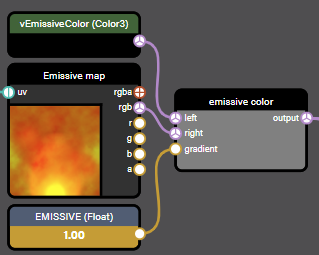If `EMISSIVE` is set to 0, the output is `vEmissiveColor`, else it is the color from the emissive map. In effect, the `EMISSIVE` boolean lets you choose to use either the constant `vEmissiveColor` color or the color from the texture map as the emissive color.

#### Discarding the fragment based on alpha cutoff value

This construct is meant to discard the fragment if alpha testing is enabled and if the alpha value is below some threshold value (cutoff value). It looks like this: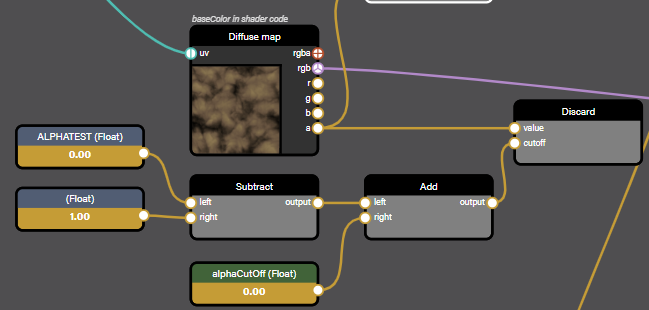As you can see, the `alphaCutOff` node is not directly connected to the `cutoff` input of Discard (the Discard block will discard the fragment if the `value` input is lower than the `cutoff` input). That's because we need to let the user enable or disable this feature.

What it does instead is comparing the alpha value from the diffuse texture to `ALPHATEST - 1 + alphaCutOff`, `ALPHATEST` being the boolean value that lets the user enable (1) or disable (0) the feature.

If `ALPHATEST = 1`, the computed value is `alphaCutOff`, which is the expected input for `Discard.cutoff` in that case (alpha testing is enabled).

If `ALPHATEST = 0`, the computed value is `-1 + alphaCutOff`. As `alphaCutOff` is a value between 0 and 1, `-1 + alphaCutOff` will always be lower or equal to 0. So, `Discard.cutoff` <= 0 in that case, meaning the fragment will never be discarded (which is the expected result when alpha testing is disabled).

You could also have used `Lerp(0, alphaCutOff, ALPHATEST)` as the input for `Discard.cutoff`, but it's likely that the addition + subtraction used above is faster than a `Lerp` on GPUs (would need some benchmarking to be sure), even if it's by a small (negligible) margin.

## Creating PBR materials

You can use those playgrounds and materials as starting points for your own experiments to create PBR materials in the NME (note that the node material may take some time to load in the PG - the mesh will stay black until the material is loaded):

• Full use of all PBR blocks:
• PBR material with sheen only:
• PBR material with clear coat only:
• PBR material with sub surface only:

The inputs of the different PBR blocks are using the same names as in the `PBRMetallicRoughnessMaterial` class, so you can refer to this doc for explanations about them.

Some of the parameters are available as properties when clicking on the block in the NME.

For eg, for `Reflection`: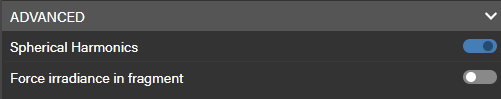Or for `PBRMetallicRoughness`: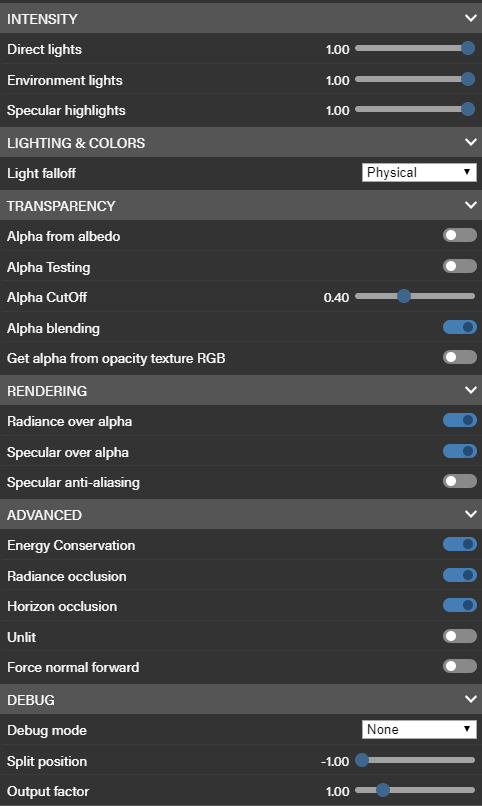As for the standard `PBRMaterial`, if no texture is provided for the Reflection / Refraction texture, the one declared at the scene level (`scene.environmentTexture`) is used instead.

By default, if something is connected to the `a` input of the `FragmentOutput` block, alpha blending is enabled. If you don't need alpha blending, don't connect this input.

Regarding the `PBRMetallicRoughness` block, you have access to each output component separately (`ambient`, `diffuse`, `specular`, ...) if you want or you can directly use `lighting` to get the composite output. In the names of the separate outputs, `dir` means `direct` (component from direct lights) and `Ind` means `Indirect` (component from indirect lighting, meaning the environment).

## Creating Post Processes

Starting with Babylon.js v4.2, you can now create post processes with the node material editor.

You need simply to change the mode to Post Process: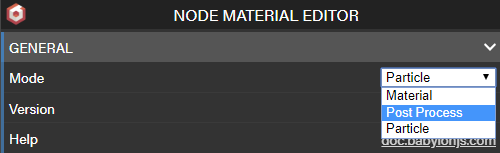In this mode, the special block CurrentScreen corresponds to the frame buffer that will be passed to your post process when you use this material as a post process in a real scenario. You can load any texture you want, it's simply an helper for you to see how your post process will render in the end.

Some blocks are made unavailable in this mode (they are hidden from the block list), as they have no meaning: the mesh, particle and animation blocks.

When you have created your post process material in the NME, you can create a regular `BABYLON.PostProcess` instance by calling the `NodeMaterial.createPostProcess` method:

``````const postProcess = nodeMaterial.createPostProcess(camera);
``````

You can also update an existing post process:

``````const myPostProcess = new BABYLON.PostProcess(...);
...
nodeMaterial.createEffectForPostProcess(myPostProcess);
``````

As for regular node materials, you can access the blocks programmatically and change their values:

Base material: https://playground.babylonjs.com/#WB27SW#4 -

Programmatically updated material: https://playground.babylonjs.com/#WB27SW#3 -

## Creating Procedural textures

Starting with Babylon.js v4.2, you can now create procedural textures with the node material editor.

You need simply to change the mode to Procedural texture this time:

Some blocks are made unavailable in this mode (they are hidden from the block list), as they have no meaning: the mesh, particle and animation blocks.

When you have created your procedural texture in the NME, you can create a regular `BABYLON.ProceduralTexture` instance by calling the `NodeMaterial.createProceduralTexture` method:

``````const postProcess = nodeMaterial.createProceduralTexture(256);
``````

As always, you can also load the NodeMaterial from our snippet server:

``````var ground = BABYLON.MeshBuilder.CreateGround("ground", {width: 6, height: 6}, scene);

BABYLON.NodeMaterial.ParseFromSnippetAsync("#A7A3UB#1", scene).then((nodeMaterial) => {
const proceduralTexture = nodeMaterial.createProceduralTexture(256);

let mat = new BABYLON.StandardMaterial();

mat.emissiveTexture = proceduralTexture;

ground.material = mat;
});
``````

Playground: https://playground.babylonjs.com/#8S19ZC#1 -

Starting with Babylon.js v4.2, you can now create particle shaders (to be used with a particle system) with the node material editor.

You need simply to change the mode to Particle: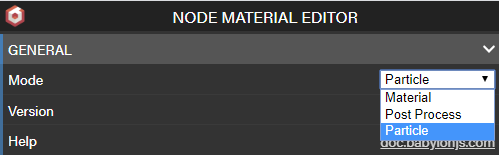Some blocks are made unavailable in this mode (they are hidden from the block list), as they have no meaning: the mesh, post process and animation blocks.

Also, in this mode, you can't create a vertex shader, only a fragment shader: the vertex shader is fixed and not updatable.

Note that everything is driven by the parameters of the particle system instance. For eg, if the current particle system displayed in the preview area does not use "ramp gradients", the `ParticleRampGradient` block does nothing (it does not add ramp gradients to the shader), it's just a pass-through. Same thing for the `ParticleBlendMultiply` block. The exception is the `ParticleTexture` block, and only in the preview area of the node editor: if you provide a texture for this block it will be used as the particle texture in the preview area, else the texture defined in the current particle system will be used. In any case, when using the material in a live program, the texture will always be the one defined by the `ParticleSystem.particleTexture` property.

The materials you create in the Particle mode can also be used for GPU particle systems, save for these restrictions:

• GPU particle systems don't support ramp gradients, so the `RampGradientBlock` won't do anything (you can still use it in your material, it will simply do nothing)
• GPU particle systems don't support the `textureMask` property, so you should not use the `ParticleTextureMask` block in your materials targeted for GPU particle systems, else display artifacts will appear

When you have created your particle shader in the NME, you can link the material to a particle system instance by calling the `NodeMaterial.createEffectForParticles` method:

``````nodeMaterial.createEffectForParticles(particleSystem);
``````

The full fragment shader used by default by the particle system can be recreated in the NME: https://nme.babylonjs.com/#X3PJMQ#1

As explained above, if you want to use this material for GPU particle systems, you should remove the use of the `ParticleTextureMask` block: https://nme.babylonjs.com/#X3PJMQ#2

You can directly setup a Node Material from a file saved from the Node Material Editor.

Here is the code to use:

``````nodeMaterial.loadAsync("file-url.json").then(() => {
nodeMaterial.build(true);
});
``````

## Sharing unique URLs

When using https://nme.babylonjs.com, you can have an additional option to save your work using a unique URL (like the Playground for instance). You can then share these urls (which are immutable).

You can use the following code to load a saved node material from a unique URL:

``````BABYLON.NodeMaterial.ParseFromSnippetAsync("2F999G", scene).then(nodeMaterial => {
sphere.material = nodeMaterial;
});
``````

When using NME within the Playground, you have the opportunity to edit your material with the Inspector and let the Playground update your snipped id automatically (each time NME will save a new snippet id it will inform the playground to also change the id in your code.

And to begin with you can simply call this code to start a new Node Material from scratch:

``````BABYLON.NodeMaterial.ParseFromSnippetAsync("_BLANK", scene).then(nodeMaterial => {
sphere.material = nodeMaterial;
});
``````

And then from there you could have used the Inspector to edit it (The inspector can replace the __BLANK with the right id later on).

## Node material examples

Here are some node material examples that you can use "as is" or extend with the NME:

## Going further

Here is a list of tutorials and informational videos about Node Material: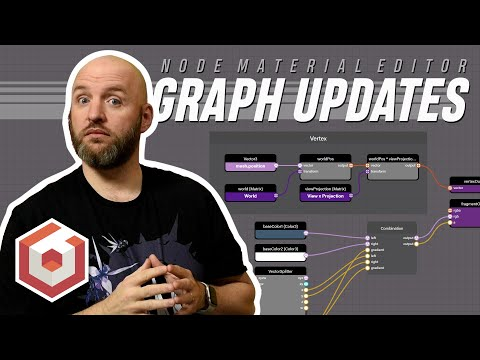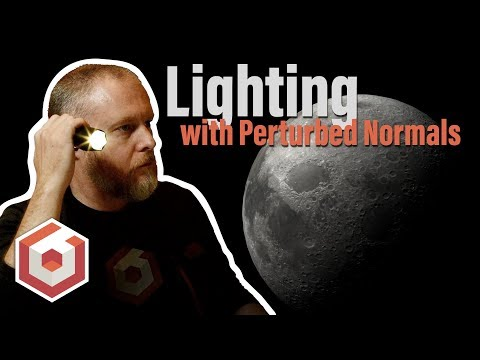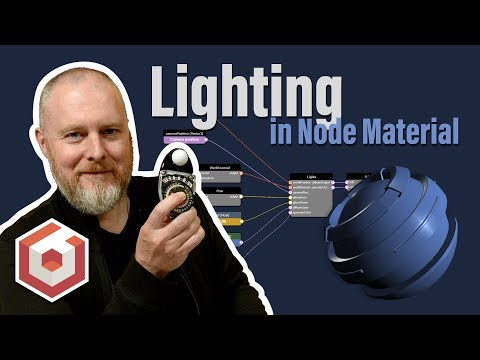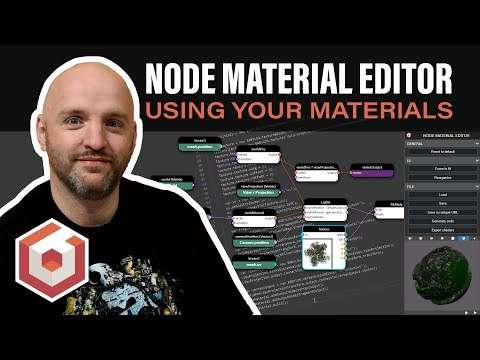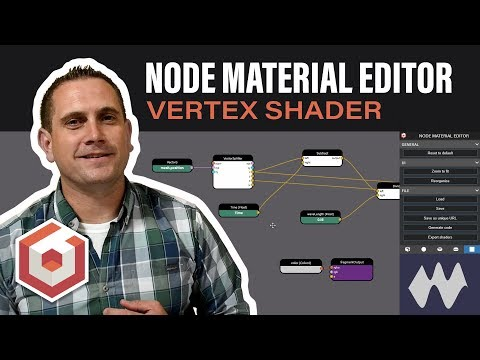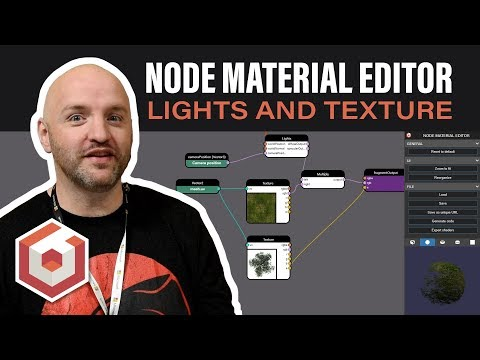You can also visit the dedicated forum topic: https://forum.babylonjs.com/t/node-materials-examples/6048